GeeksforGeeks App
Open AppBrowser
Continue

# Adding value labels on a Matplotlib Bar Chart

Prerequisites: Matplotlib

In this article, we are going to Add value labels on a Matplotlib Bar Chart. Bar Chart is the graphical display of data using bars of different heights.  We can compare different data’s using this bar chart. For plotting the data in Python we use bar() function provided by Matplotlib Library in this we can pass our data as a parameter to visualize, but the default chart is drawn on the given data doesn’t contain any value labels on each bar of the bar chart, since the default bar chart doesn’t contain any value label of each bar of the bar chart it is difficult to analyze the exact value represented by the individual bars of the bar chart.

For Plotting the bar chart with value labels we are using mainly two methods provided by Matplotlib Library.

• For making the Bar Chart
`Syntax: plt.bar(x, height, color)`
• For adding text on the Bar Chart
`Syntax: plt.text(x, y, s, ha, Bbox)`

Steps Needed:

• Import the library.
• Create the function which can add the value labels by taking x and y as a parameter, now in the function, we will run the for loop for the length of the x value we can find the length by using the len() function, and in that passed variable whose length we want.
• Now use plt.text() function to add value labels to the bar chart in this pass the x and y coordinates which will be i and y[i] which is nothing but the height of the bar and pass y[i] this represents the string which will be displayed on the given co-ordinates i.e, i and y[i].
• For adding the value labels in the center of the height of the bar just we have to divide the y co-ordinates by 2 i.e, y[i]//2 by doing this we will get the center coordinates of each bar as soon as the for loop runs for each value of i.
• With this, we can also set the parameter ha and Bbox that are horizontal alignment which aligns the value labels at the center of the bar when we pass ha=center and Bbox which display the value labels in the covered rectangular box for this we have to create a dictionary using dict() and in this pass the facecolor that is color we want and alpha if we want the opacity we can set.
• Now after creating the function we have to create main function from where the program starts to run.
• Create or import the data for which bar chart is plotted.
• Give the figure size so that the values and labels of the plot clearly visible and cannot be overlapping you can set accordingly by using plt.figure() function in which pass the figsize as a parameter.
• Now plot the bar chart using plt.bar() function in which pass the data values and color accordingly if you want or the default color will be displayed.
• Now after making the bar chart call the function which we had created for adding value labels.
• Set the title, X-axis labels and Y-axis labels of the chart/plot.
• Now visualize the plot by using plt.show() function.

Example 1: Adding value labels on the Bar Chart at the default setting.

## Python

 `# importing library``import` `matplotlib.pyplot as plt` `# function to add value labels``def` `addlabels(x,y):``    ``for` `i ``in` `range``(``len``(x)):``        ``plt.text(i,y[i],y[i])` `if` `__name__ ``=``=` `'__main__'``:``    ``# creating data on which bar chart will be plot``    ``x ``=` `[``"Engineering"``, ``"Hotel Management"``, ``"MBA"``,``         ``"Mass Communication"``, ``"BBA"``, ``"BSc"``, ``"MSc"``]``    ``y ``=` `[``9330``, ``4050``, ``3030``, ``5500``,``         ``8040``, ``4560``, ``6650``]``    ` `    ``# making the bar chart on the data``    ``plt.bar(x, y)``    ` `    ``# calling the function to add value labels``    ``addlabels(x, y)``    ` `    ``# giving title to the plot``    ``plt.title(``"College Admission"``)``    ` `    ``# giving X and Y labels``    ``plt.xlabel(``"Courses"``)``    ``plt.ylabel(``"Number of Admissions"``)``    ` `    ``# visualizing the plot``    ``plt.show()`

Output: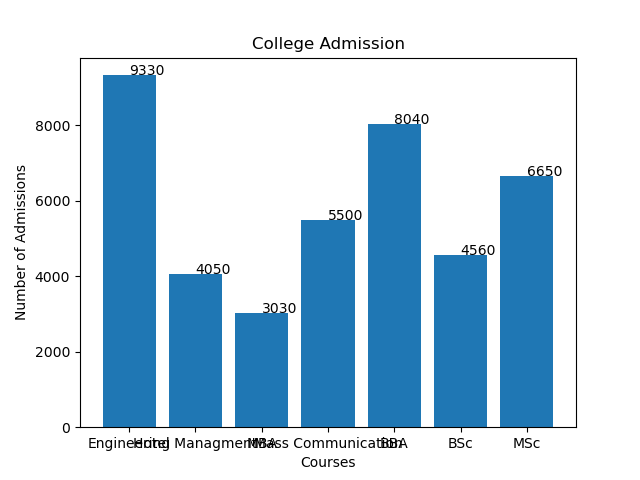The above plot is plotted on the default settings, from the above figure we can observe that the value labels for each bar is added on the top, but they are present slightly on the right side of the top of the bar and on the X axis some names of courses are overlapping.

Example 2: Adding value labels in the center of each Bar on the Bar Chart.

## Python

 `# importing library``import` `matplotlib.pyplot as plt` `# function to add value labels``def` `addlabels(x,y):``    ``for` `i ``in` `range``(``len``(x)):``        ``plt.text(i, y[i], y[i], ha ``=` `'center'``)` `if` `__name__ ``=``=` `'__main__'``:``  ` `    ``# creating data on which bar chart will be plot``    ``x ``=` `[``"Engineering"``, ``"Hotel Management"``,``         ``"MBA"``, ``"Mass Communication"``, ``"BBA"``,``         ``"BSc"``, ``"MSc"``]``    ``y ``=` `[``9330``, ``4050``, ``3030``, ``5500``,``         ``8040``, ``4560``, ``6650``]``    ` `    ``# setting figure size by using figure() function``    ``plt.figure(figsize ``=` `(``10``, ``5``))``    ` `    ``# making the bar chart on the data``    ``plt.bar(x, y)``    ` `    ``# calling the function to add value labels``    ``addlabels(x, y)``    ` `    ``# giving title to the plot``    ``plt.title(``"College Admission"``)``    ` `    ``# giving X and Y labels``    ``plt.xlabel(``"Courses"``)``    ``plt.ylabel(``"Number of Admissions"``)``    ` `    ``# visualizing the plot``    ``plt.show()`

Output: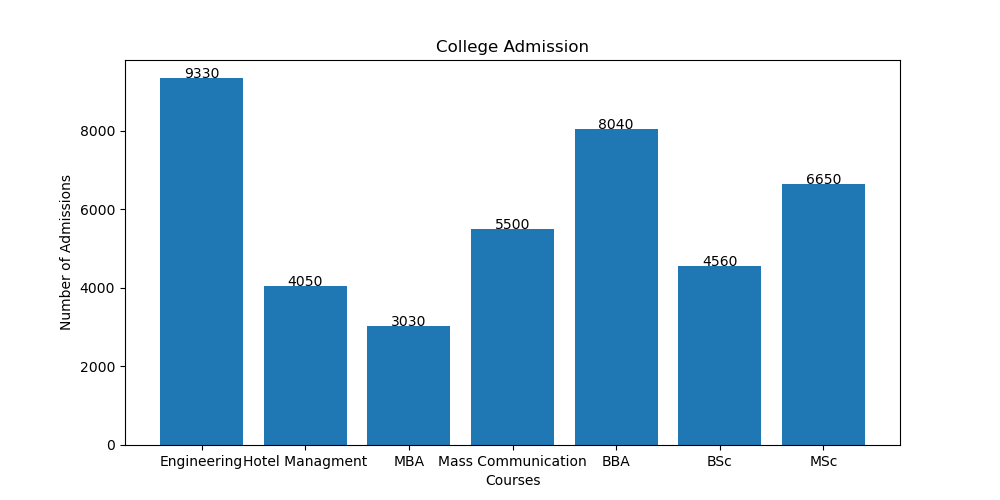In the above plot, we can observe that the value labels are aligned at the center top of each bar and on the X-axis the name of the courses which are overlapping are also shown separately.

For aligning the value labels in the center we had passed only one parameter in the plt.text() function which is “ha=’center” that is the horizontal alignment of the text, and for showing the names of the courses separately we had added plt.figure() function before making the bar chart in which we had passed the figure size as a parameter and rest of the code is same.

Example 3: Adding value labels by putting them in a rectangular box.

## Python

 `# importing library``import` `matplotlib.pyplot as plt` `# function to add value labels``def` `addlabels(x,y):``    ``for` `i ``in` `range``(``len``(x)):``        ``plt.text(i, y[i], y[i], ha ``=` `'center'``,``                 ``Bbox ``=` `dict``(facecolor ``=` `'red'``, alpha ``=``.``8``))` `if` `__name__ ``=``=` `'__main__'``:``  ` `    ``# creating data on which bar chart will be plot``    ``x ``=` `[``"Engineering"``, ``"Hotel Management"``,``         ``"MBA"``, ``"Mass Communication"``, ``"BBA"``, ``"BSc"``, ``"MSc"``]``    ``y ``=` `[``9330``, ``4050``, ``3030``, ``5500``, ``8040``, ``4560``, ``6650``]``    ` `    ``# setting figure size by using figure() function``    ``plt.figure(figsize ``=` `(``10``,``5``))``    ` `    ``# making the bar chart on the data``    ``plt.bar(x, y)``    ` `    ``# calling the function to add value labels``    ``addlabels(x, y)``    ` `    ``# giving title to the plot``    ``plt.title(``"College Admission"``)``    ` `    ``# giving X and Y labels``    ``plt.xlabel(``"Courses"``)``    ``plt.ylabel(``"Number of Admissions"``)``    ` `    ``# visualizing the plot``    ``plt.show()`

Output: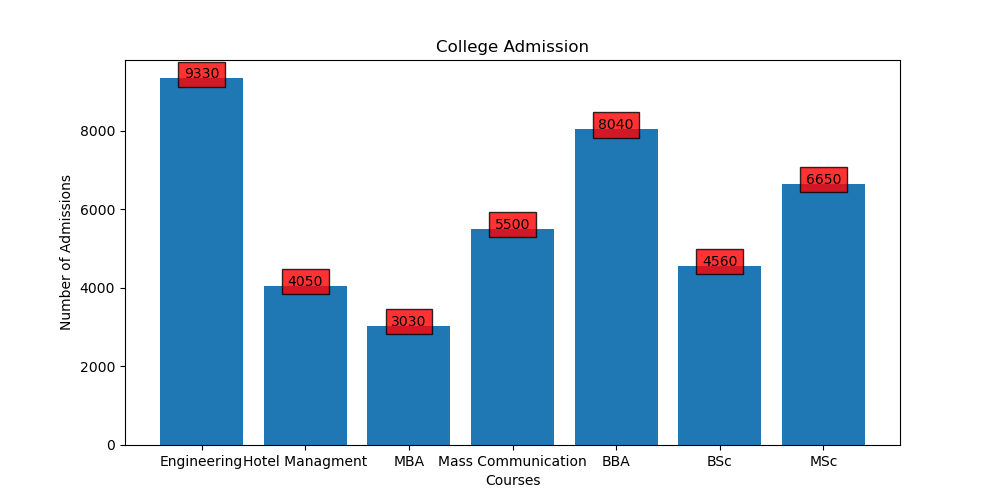In the above example, we had added the value label which is covered in the rectangular box, for this in the plt.text() function we have to pass the parameter Bbox in which we have to create the dictionary and in that dictionary, we can give facecolor of our choice and alpha which gives the opacity to the box which we can set accordingly.

Example 4: Adding value labels at the center of the height of each bar of the Bar Chart.

## Python

 `# importing library``import` `matplotlib.pyplot as plt` `# function to add value labels``def` `addlabels(x,y):``    ``for` `i ``in` `range``(``len``(x)):``        ``plt.text(i, y[i]``/``/``2``, y[i], ha ``=` `'center'``)` `if` `__name__ ``=``=` `'__main__'``:``  ` `    ``# creating data on which bar chart will be plot``    ``x ``=` `[``"Engineering"``, ``"Hotel Management"``, ``"MBA"``,``         ``"Mass Communication"``, ``"BBA"``, ``"BSc"``, ``"MSc"``]``    ` `    ``y ``=` `[``9330``, ``4050``, ``3030``, ``5500``,``         ``8040``, ``4560``, ``6650``]``    ` `    ``# setting figure size by using figure() function``    ``plt.figure(figsize ``=` `(``10``,``5``))``    ` `    ``# making the bar chart on the data``    ``plt.bar(x, y)``    ` `    ``# calling the function to add value labels``    ``addlabels(x, y)``    ` `    ``# giving title to the plot``    ``plt.title(``"College Admission"``)``    ` `    ``# giving X and Y labels``    ``plt.xlabel(``"Courses"``)``    ``plt.ylabel(``"Number of Admissions"``)``    ` `    ``# visualizing the plot``    ``plt.show()`

Output: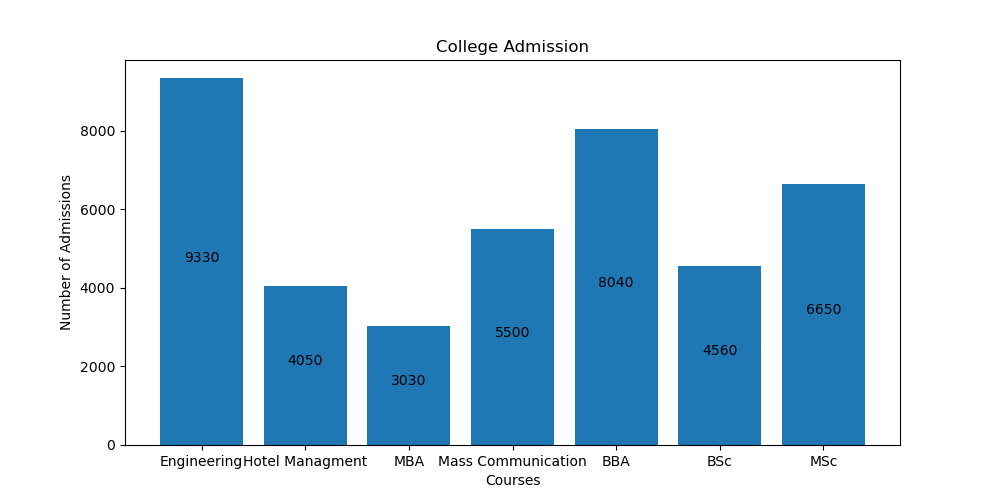In the above example for adding the value label in the center of the height of each bar, we had just divided the y coordinates by 2 in the plt.text() function inside the for a loop.

Example 5: Adding the value labels covered in rectangular box at the center of the height of each bar of Bar Chart.

## Python

 `# importing library``import` `matplotlib.pyplot as plt` `# function to add value labels``def` `addlabels(x,y):``    ``for` `i ``in` `range``(``len``(x)):``        ``plt.text(i, y[i]``/``/``2``,y[i], ha ``=` `'center'``,``                 ``Bbox ``=` `dict``(facecolor ``=` `'white'``, alpha ``=` `.``5``))` `if` `__name__ ``=``=` `'__main__'``:``  ` `    ``# creating data on which bar chart will be plot``    ``x ``=` `[``"Engineering"``, ``"Hotel Management"``, ``"MBA"``,``         ``"Mass Communication"``, ``"BBA"``, ``"BSc"``, ``"MSc"``]``    ``y ``=` `[``9330``, ``4050``, ``3030``, ``5500``,``         ``8040``, ``4560``, ``6650``]``    ` `    ``# setting figure size by using figure() function``    ``plt.figure(figsize ``=` `(``10``,``5``))``  ` `    ``# making the bar chart on the data with color red``    ``plt.bar(x, y, color ``=` `'red'``)``    ` `    ``# calling the function to add value labels``    ``addlabels(x, y)``    ` `    ``# giving title to the plot``    ``plt.title(``"College Admission"``)``    ` `    ``# giving X and Y labels``    ``plt.xlabel(``"Courses"``)``    ``plt.ylabel(``"Number of Admissions"``)``    ` `    ``# visualizing the plot``    ``plt.show()`

Output: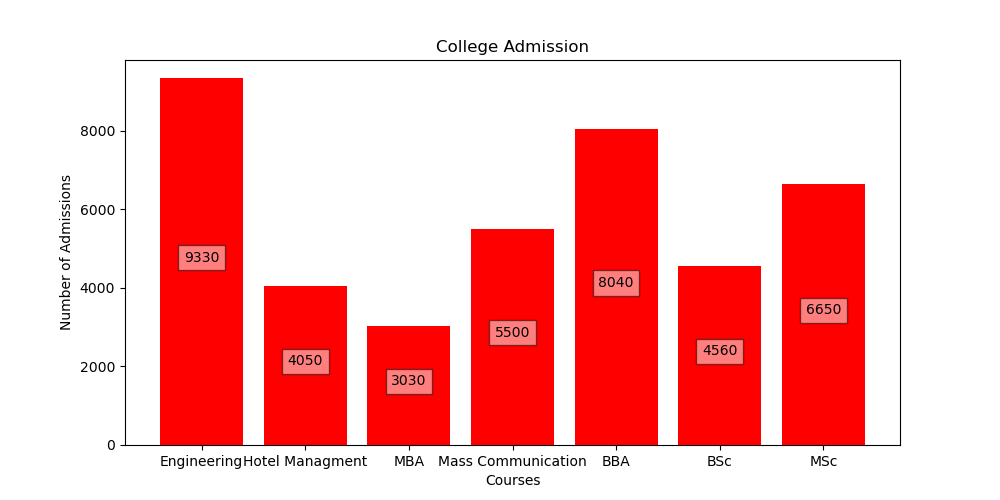In the above example, we had done the same thing as we had done in example 3 but the only difference is we had divided the coordinates of y by 2 so that the value labels should be displayed at the center of the height of the bar and also we had changed the color of the bar chart to red by passing the color parameter in the plt.bar() function.

My Personal Notes arrow_drop_up